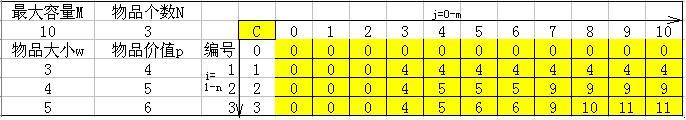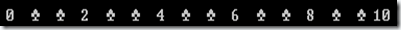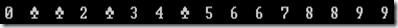基础算法系列总结：动态规划(解公司外包成本问题）

动态规划算法通常用于求解具有某种最优性质的问题。在这类问题中，可能会有许多可行解。每一个解都对应于一个值，我们希望找到具有最优值的解。动态规划算法与分治法类似，其基本思想也是将待求解问题分解成若干个子问题，先求解子问题，然后从这些子问题的解得到原问题的解。与分治法不同的是，适合于用动态规划求解的问题，经分解得到子问题往往不是互相独立的。若用分治法来解这类问题，则分解得到的子问题数目太多，有些子问题被重复计算了很多次。如果我们能够保存已解决的子问题的答案，而在需要时再找出已求得的答案，这样就可以避免大量的重复计算，节省时间。我们可以用一个表来记录所有已解的子问题的答案。不管该子问题以后是否被用到，只要它被计算过，就将其结果填入表中。这就是动态规划法的基本思路。具体的动态规划算法多种多样，但它们具有相同的填表格式。

上面这段定义文字是我Copy过来的，目前我还没有那么好的总结能力，呵呵，如果大家对于动态规划不是很熟悉的话，可能看着上面一段文字会不知所云，我一般的学习方法是首先扫描一下基本定义，不深究（有点不求甚解的味道），然后去看一些实例，结合自己的体会，最后再回顾，精读一下定义，这样我对定义才能够真正的理解。下面我们依托一个经典的算法问题来体现上面这段文字的思想，0-1背包问题在算法学习中可谓是必修课程，一般在讲动态规划问题的时候都会用到这个例子。

M,N

W1,P1

W2,P2

......

f[i][j] = max{f[i-1][j], f[i-1][j-w[i]]+P[i]}

10,3
3,4
4,5
5,6View Code
#include<stdio.h>
int c;
int knapsack(int m,int n)
{

int i,j,w,p;

for(i=1;i<n+1;i++)
scanf(
"\n%d,%d",&w[i],&p[i]);

for(i=0;i<10;i++)

for(j=0;j<100;j++)
c[i][j]
=0;

for(i=1;i<n+1;i++)

for(j=1;j<m+1;j++)
{

if(w[i]<=j)
{

if(p[i]+c[i-1][j-w[i]]>c[i-1][j])
c[i][j]
=p[i]+c[i-1][j-w[i]];

else
c[i][j]
=c[i-1][j];
}

else c[i][j]=c[i-1][j];
}

return(c[n][m]);

}
int main()
{

int m,n;int i,j;
scanf(
"%d,%d",&m,&n);
printf(
"Input each one:\n");
printf(
"%d",knapsack(m,n));
printf(
"\n");

for(i=0;i<n+1;i++)

for(j=0;j<m+1;j++)
{
printf(
"%2d ",c[i][j]);

if(j==m)printf("\n");
}
}

Zealot Yin 所在的X公司需要至少W个其他公司提供的外包人员，现在有N家公司向X公司提供了可选方案，其中

P_i代表可提供外包人员单位数，如5人为一个单位数，若选用该公司方案，则必须采用整单位数的人数，如5人为一个单位数，则X公司只能采用n*5个人数（n=0,1,2,….)。C_i代表为P_i单位数员工提供的总工资，单位是万元

N     W

P_i  C_i

2 15
3 2
5 3View Code
#include <stdio.h>
#define MAX 1000000000

int dp;

int min(int x,int y) { return x<y?x:y; }

int main()
{

int n,h;

int i,j;

int p,c;

while(scanf("%d%d",&n,&h)==2)
{
dp[
0] = 0;

for ( i = 1 ; i <= h ; i ++)
{
dp[i]
= MAX;
}

for( i = 0 ; i < n ; i++)
{
scanf(
"%d%d",&p,&c);

for(j = 0 ; j<=h ;j++
{

if( j + p > h )  dp[h] = min (dp[h] , dp[j] + c );

else     dp[ j + p ] = min ( dp[j+p] , dp[j] + c );

char disstr="x";
printf(
"\n");

for(int x=0;x<=h;x++)
{
if(dp[x]==1000000000)
printf(
"%2c ",disstr);

else
printf(
"%2d ",dp[x]);
}
}
printf(
"\n");
}

printf(
"The Min Value Is %d\n",dp[h]);
}

return 0;
}

动态规划算法的基本思想是：将待求解的问题分解成若干个相互联系的子问题，先求解子问题，然后从这些子问题的解得到原问题的解；对于重复出现的子问题，只在第一次遇到的时候对它进行求解，并把答案保存起来，让以后再次遇到时直接引用答案，不必重新求解。动态规划算法将问题的解决方案视为一系列决策的结果，与贪婪算法不同的是，在贪婪算法中，每采用一次贪婪准则，便做出一个不可撤回的决策；而在动态规划算法中，还要考察每个最优决策序列中是否包含一个最优决策子序列，即问题是否具有最优子结构性质。

算法系列目录：

1.递归与分治

posted @ 2011-05-17 01:31  大熊先生|互联网后端技术  阅读(7082)  评论(10编辑  收藏  举报